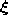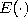Next: Feature Mapping Up: Goals of Competitive Learning Previous: Error Minimization

# Entropy Maximization

Sometimes the reference vectors should be distributed such that each reference vector has the same chance to be winner for a randomly generated input signal:If we interpret the generation of an input signal and the subsequent mapping onto the nearest unit inas random experiment which assigns a valueto the random variable X, then (3.3) is equivalent to maximizing the entropywithbeing the expectation operator.

If the data is generated from a continuous probability distribution, then (3.3) is equivalent toIn the case of a finite data set(3.3) corresponds to the situation where each Voronoi setcontains (up to discretization effects) the same number of data vectors:An advantage of choosing reference vectors such as to maximize entropy is the inherent robustness of the resulting system. The removal (or ``failure'') of any reference vector affects only a limited fraction of the data.

Entropy maximization and error minimization can in general not be achieved simultaneously. In particular if the data distribution is highly non-uniform both goals differ considerably. Consider, e.g., a signal distributionwhere 50 percent of the input signals come from a very small (point-like) region of the input space, whereas the other fifty percent are uniformly distributed within a huge hypercube. To maximize entropy half of the reference vectors have to be positioned in each region. To minimize quantization error however, only one single vector should be positioned in the point-like region (reducing the quantization error for the signals there basically to zero) and all others should be uniformly distributed within the hypercube.

Bernd Fritzke
Sat Apr 5 18:17:58 MET DST 1997